Courses

# 28 Year NEET Questions: Electrostatics Potential and Capacitance

## 36 Questions MCQ Test Physics 28 Years Past year papers for NEET/AIPMT Class 12 | 28 Year NEET Questions: Electrostatics Potential and Capacitance

Description
This mock test of 28 Year NEET Questions: Electrostatics Potential and Capacitance for NEET helps you for every NEET entrance exam. This contains 36 Multiple Choice Questions for NEET 28 Year NEET Questions: Electrostatics Potential and Capacitance (mcq) to study with solutions a complete question bank. The solved questions answers in this 28 Year NEET Questions: Electrostatics Potential and Capacitance quiz give you a good mix of easy questions and tough questions. NEET students definitely take this 28 Year NEET Questions: Electrostatics Potential and Capacitance exercise for a better result in the exam. You can find other 28 Year NEET Questions: Electrostatics Potential and Capacitance extra questions, long questions & short questions for NEET on EduRev as well by searching above.
QUESTION: 1

### A 4μF conductor is charged to 400 volts andthen its plates are joined through a resistance of1 kΩ. The heat produced in the resistance is    

Solution:

The energy stored in the capacitor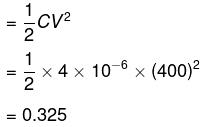This energy will be converted into heat in the resistor

QUESTION: 2

### A hollow metal sphere of radius 10 cm is chargedsuch that the potential on its surface is 80 V. Thepotential at the centre of the sphere is 

Solution:

Potential at the centre of the sphere
= potential on the surface = 80 V.

QUESTION: 3

### The four capacitors, each of 25μ F are connected as shown in fig. The dc voltmeter reads 200 V. The charge on each plate of capacitor is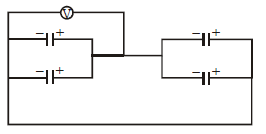Solution:

Charge on each plate of each capacitor

Q =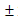CV =25 x10-6 x 200
=5 x10-3C

QUESTION: 4

If the potential of a capacitor having capacity 6μF is increased from 10 V to 20 V, then increase in its energy will be     

Solution:

Capacitance of capacitor (C) = 6μF = 6 ×10–6 F; Initial potential (V1) = 10 V and final potential (V2) = 20 V.
The increase in energy (ΔU)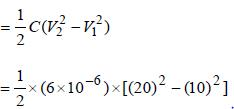= (3x10-6 )x300 = 9x10-4 J .

QUESTION: 5

A parallel plate condenser with oil between the plates (dielectric constant of oil K = 2) has a capacitance C. If the oil is removed, then capacitance of the capacitor becomes

Solution:

When oil is placed between space of plates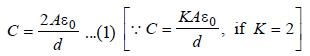When oil is removed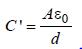... (2)

On comparing both equations, we get
C ' = C/2

QUESTION: 6

What is the effective capacitance between points X and Y?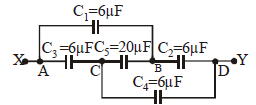Solution: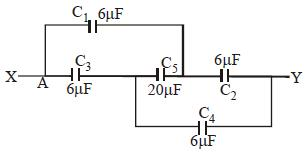Equivalent circuit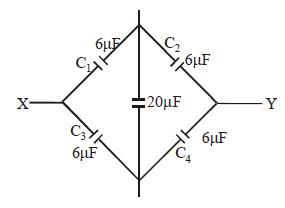Here,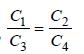Hence, no charge will flow through 20μF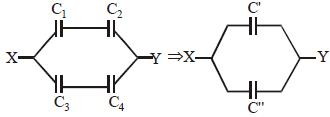C1 and C2 are in series, also C3 and C4 are in
series.
Hence, C' = 3 μF, C'' = 3 μF
C' and C'' are in parallel.
Hence net capacitance = C' + C'' = 3 + 3
= 6 μF

QUESTION: 7

A capacitor is charged to store an energy U.The charging battery is disconnected. Anidentical capacitor is now connected to the firstcapacitor in parallel. The energy in each of thecapacitor is 

Solution:

Energy stored in a capacitor is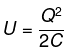As the battery is disconnected, total charge Q is shared equally by two capacitors.
So energy of each capacitor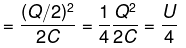QUESTION: 8

In a parallel plate capacitor, the distance between the plates is d and potential difference across the plates is V. Energy stored per unit volume between the plates of capacitor is

Solution:

Energy stored per unit volume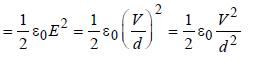(∵ E = V/d)

QUESTION: 9

The capacity of a parallel plate condenser is 10μF when the distance between its plates is 8cm. If the distance between the plates is reducedto 4 cm then the capacity of this parallel platecondenser will be 

Solution:

C = 10 μF          d = 8 cm
C ' = ?                d ' = 4 cm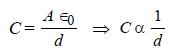If d is halved then C will be doubled.

Hence, C ' = 2C = 2 × 10μF = 20μF

QUESTION: 10

Energy stored in a capacitor is

Solution:

Energy stored in capacitor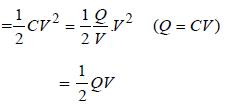QUESTION: 11

A capacitor C1 is charged to a potential difference V. The charging battery is then removed and the capacitor is connected to an uncharged capacitor C2. The potential difference across the combination is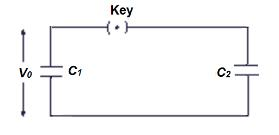Solution:

Charge Q = C1V
Total capacity of combination (parallel)

C = C1+ C2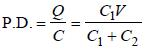QUESTION: 12

A solid spherical conductor is given a charge. The electrostatic potential of the conductor is      

Solution:

Electric potential is constant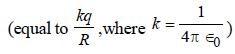within or on the surface of conductor.

QUESTION: 13

Each corner of a cube of side l has a negative charge, –q. The electrostatic potential energy of a charge q at the centre of the cube is    

Solution:

Length of body diagonal =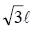∴ Distance of centre of cube from each
corner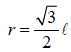P.E at centre
= 8 × Potential Energy due to A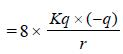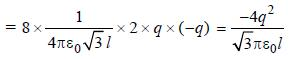QUESTION: 14

Three capacitors each of capacity 4μF are to be connected in such a way that the effective capacitance is 6 μF. This can be done by       

Solution:

For series,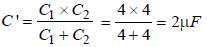For parallel, Ceq = C '+C3 = 2 + 4 = 6μF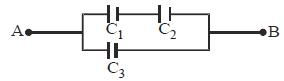QUESTION: 15

An electric dipole has the magnitude of its chargeas q and its dipole moment is p. It is placed inuniform electric field E. If its dipole moment is along the direction of the field, the force on itand its potential energy are respectively               

Solution:

When the dipole is in the direction of field then net force is qE + (–qE) = 0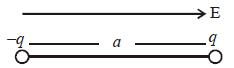and its potential energy is minimum
= – P.E. = –qaE

QUESTION: 16

A bullet of mass 2 g is having a charge of 2μC.Through what potential difference must it beaccelerated, starting from rest, to acquire a speedof 10 m/s?          

Solution: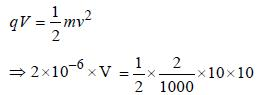∴ V = 50 kV

QUESTION: 17

As per the diagram, a point charge +q is placed at the origin O. Work done in taking another point charge –Q from the point A [coordinates (0, a)] to another point B [coordinates (a, 0)] along the straight path AB is: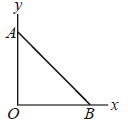Solution:

We know that potential energy of two charge system is given by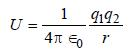According to question,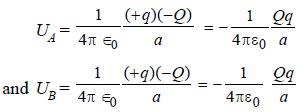ΔU= UB–UA = 0
We know that for conservative force,
W = –ΔU = 0

QUESTION: 18

Two charges q1 and q2 are placed 30 cm apart, as shown in the figure. A third charge q3 is moved along the arc of a circle of radius 40 cm from C to D. The change in the potential energy of the system is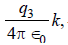where k is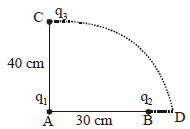Solution:

We know that potential energy of discrete system of charges is given by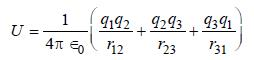According to question,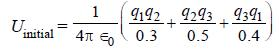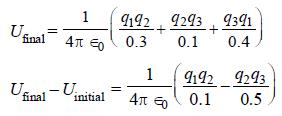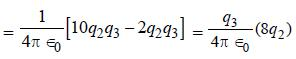QUESTION: 19

A network of four capacitors of capacity equal to C1 = C, C2 = 2C, C3 = 3C and C4 = 4C are conducted to a battery as shown in the figure. The ratio of the charges on C2 and C4 is: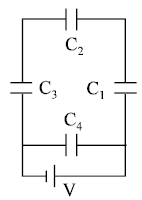Solution: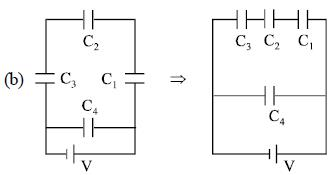Equivalent capacitance for three capacitors (C1, C2 & C3) in series is given by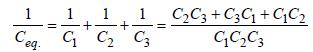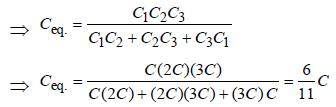⇒ Charge on capacitors (C1, C2 & C3) in series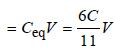Charge on capacitor C4 = C4V = 4C V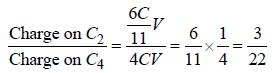QUESTION: 20

A parallel plate air capacitor is charged to apotential difference of V volts. After disconnecting the charging battery the distance between the plates of the capacitor is increased using an insulating handle. As a result the potential difference between the plates    

Solution:

If we increase the distance between the plates its capacity decreases resulting in higher potential as we know Q = CV. Since Q is constant (battery has been disconnected), on decreasing C, V will increase

QUESTION: 21

Charges +q and –q are placed at points A and B respectively which are a distance 2L apart, C is the midpoint between A and B. The work done in moving a charge +Q along the semicircle CRD is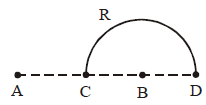Solution: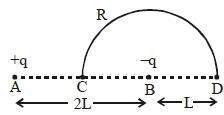Potential at C = VC = 0
Potential at D = VD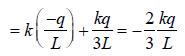Potential difference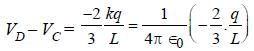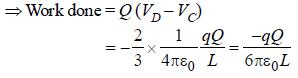QUESTION: 22

Two condensers, one of capacity C and other of capacity C/2 are connected to a V-volt battery, as shown.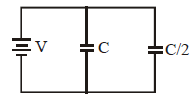The work done in charging fully both the  condensers is

Solution:

Work done = Change in energy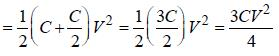QUESTION: 23

The electric potential at a point in free space due to a charge Q coulomb is Q × 1011 volts. The electric field at that point is 

Solution:

Given that, V = Q × 1011 volts
Electric potential at point is given by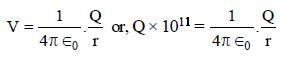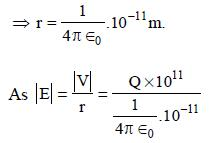= 4π∈0 Q x 1022 volt m-1

QUESTION: 24

The energy required to charge a parallel plate condenser of plate separation d and plate area of cross-section A such that the uniform electric field between the plates is E, is 

Solution:

The energy required to charge a parallel
plate condenser is given by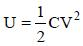As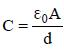and V = E.d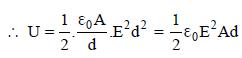QUESTION: 25

Three concentric spherical shells have radii a, b and c (a < b < c) and have surface charge densities σ , – σ and σ respectively. If VA, VB and VC denotes the potentials of the three shells, then for c = a + b, we have 

Solution:

c = a + b.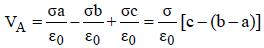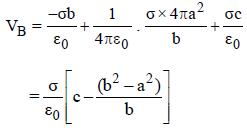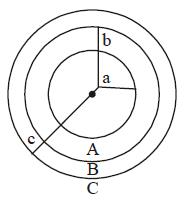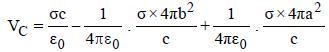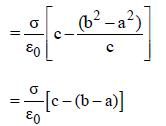VA = VC ≠ VB

QUESTION: 26

Three capacitors each of capacitance C and of breakdown voltage V are joined in series. The capacitance and breakdown voltage of the combination will be        

Solution:

In series combination of capacitors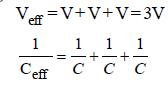Thus, the capacitance and breakdown
voltage of the combination will be C/3 and 3V

QUESTION: 27

The electric potential at a point (x, y, z) is given by V = – x2y – xz3 + 4. The electric field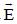at that point is   

Solution:

The electric field at a point is equal to
negative of potential gradient at that point.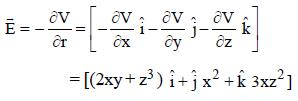QUESTION: 28

A series combination of n1 capacitors, each of value C1, is charged by a source of potential difference 4 V. When another parallel combination of n2 capacitors, each of value C2, is charged by a source of potential difference V, it has the same (total) energy stored in it, as the first combination has. The value of C2 , in terms of C1, is then   

Solution:

In series,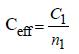∴ Energy stored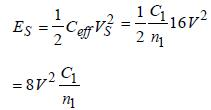In parallel, Ceff = n2 C2

∴ Energy stired ,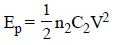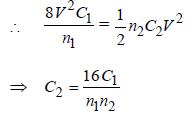QUESTION: 29

A condenser of capacity C is charged to a potential difference of V1. The plates of the condenser are then connected to an ideal inductor of inductance L. The current through the inductor when the potential difference across the condenser reduces to V2 is

Solution:

q = CV1 cos ωt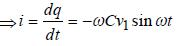Also,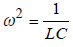and V = V1 cosωt

At t = t1 , V = V2 and i = -ωCV1 sinωt1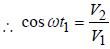(–ve sign gives direction)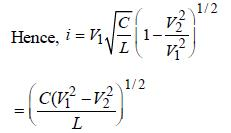QUESTION: 30

Two parallel metal plates having charges + Qand –Q face each other ata certain distance between them. If the plaves are now dipped inkerosene oil tank, the electric field between the plates will

Solution:

Electric field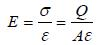ε of kerosine oil is more than that of air.
As ε increases, E decreases

QUESTION: 31

A parallel plate condenser has a uniform electric field E(V/m) in the space between the plates. If the distance between the plates is d(m) and area of each plate is A(m2) the energy (joules) stored in the condenser is 

Solution: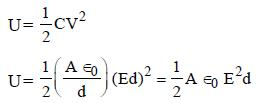QUESTION: 32

Four electric charges +q, +q, –q and – q are placed at the corners of a square of side 2L (see figure). The electric potential at point A, midway between the two charges +q and +q, is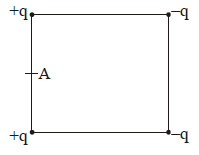Solution:

Distance of point A from the two +q
charges = L.
Distance of point A from the two –q
charges =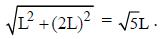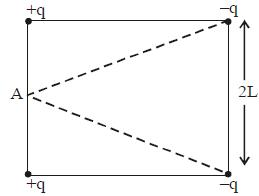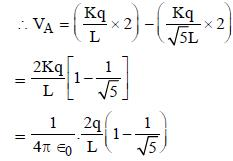QUESTION: 33

The potential energy of particle in a force field is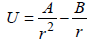, where A and B are positive constants and r is the distance of particle from the centre of the field. For stable equilibrium, the distance of the particle is : 

Solution:

for equilibrium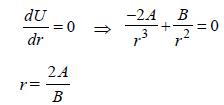for stable equilibrium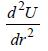should be positive for the value of r.

here,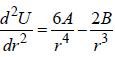is +ve value for r = 2A/B so

QUESTION: 34

Four point charges –Q, –q, 2q and 2Q are placed, one at each corner of the square. The relation between Q and q for which the potential at the centre of the square is zero is : 

Solution:

Let the side length of square be 'a' then
potential at centre O is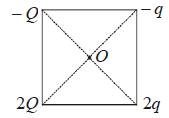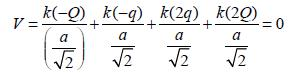(given)

= – Q – q + 2q + 2Q = 0 = Q + q = 0
= Q = – q

QUESTION: 35

A parallel plate capacitor has a uniform electric field E in the space between the plates. If the distance between the plates is d and area of each plate is A, the energy stored in the capacitor is :     [2012M]

Solution:

The energy stored by a capacitor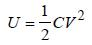...(1)

V is the p.d. between two plates of the
capacitor.
The capacitance of the parallel plate
capacitor
V = E.d.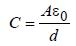Substituting the value of C in equation (i)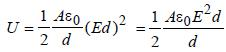QUESTION: 36

A, B and C are three points in a uniform electric field. The electric potential is [NEET 2013]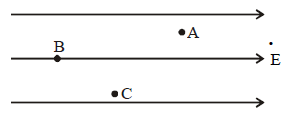Solution:

Potential at B, VB is maximum
VB > VC > VA
As in the direction of electric field
potential decreases.